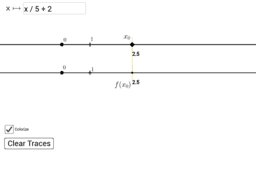# Graphs and Mapping Diagrams

Topic:
Diagrams
Visualizing Functions with Mapping Diagrams and Graphs. Functions defined on R and RP1=S1, the circle.
•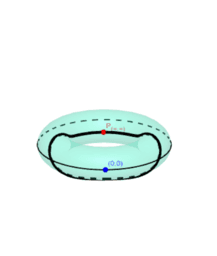### Graph of a function RP1 -> RP1

•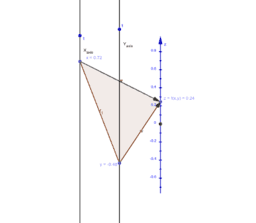### 3 Dimensional Mapping Diagram for z= f(x,y)

•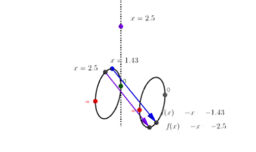### Mapping Diagram of Function : RP1 to RP1

•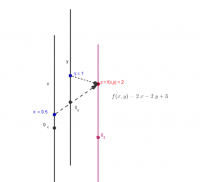### 3D Mapping Diagram for linear z=f(x,y) with focus point

•### Graph w/ Mapping diagram: Estimating The Derivative

•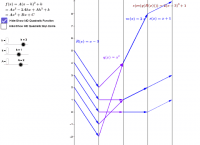### Mapping Diagrams (Real) Visualize Solving a Quadratic Equation

•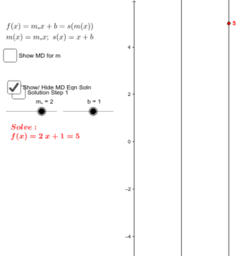### Mapping Diagrams To Visualize Solving a Linear Equation

•### Mapping Diagrams To Visualize Solving a Linear Equation

•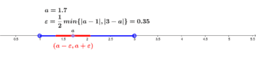### (a,b) is an open interval

•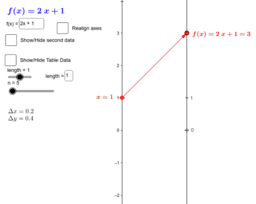### Mapping Diagram, Spreadsheet, Graph, Realign, Focus

•